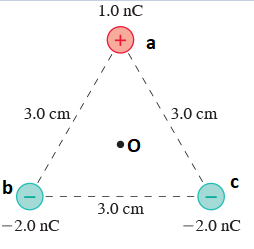# Problem: What is the electric potential at the point indicated with the dot in the figure? Express your answer to two significant figures and include the appropriate units.

###### FREE Expert Solution
83% (155 ratings)
###### FREE Expert Solution

Electric potential due to a point charge:

$\overline{){\mathbf{V}}{\mathbf{=}}\frac{\mathbf{k}\mathbf{q}}{\mathbf{r}}}$

For an equilateral triangle, the distance from the center to any vertex is given by:

$\overline{){\mathbf{r}}{\mathbf{=}}\frac{\mathbf{a}}{\sqrt{\mathbf{3}}}}$

where r = Oa = Ob = Oc and a = 3.0 cm.

83% (155 ratings)###### Problem Details

What is the electric potential at the point indicated with the dot in the figure? Express your answer to two significant figures and include the appropriate units.